Next: Reflection Coefficient Up: Wave Propagation in Inhomogeneous Previous: Ionospheric Radio Wave Propagation

# WKB Approximation

Consider a radio wave that is vertically incident, from below, on a horizontally stratified ionosphere. Because the wave normal is initially aligned along the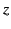-axis, and as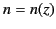, we expect all field components to be functions ofonly, so that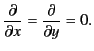(1060)

In this situation, Equations (1058)-(1061) reduce to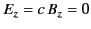, with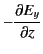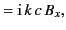(1061)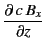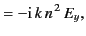(1062)

and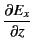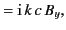(1063)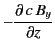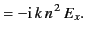(1064)

Note that Equations (1063)-(1064) and (1065)-(1066) are isomorphic and completely independent of one another. It follows that, without loss of generality, we can assume that the wave is linearly polarized with its electric vector parallel to the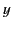-axis. In other words, we need only consider the solution of Equations (1063)-(1064). The solution of Equations (1065)-(1066) is of exactly the same form, except that it describes a linear polarized wave with its electric vector parallel to the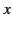-axis.

Equations (1063)-(1064) can be combined to give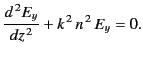(1065)

Incidentally, because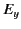is a function ofonly, we now use the total derivative sign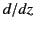, instead of the partial derivative sign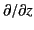. The solution of the previous equation for the case of a uniform medium, where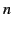is constant, is straightforward: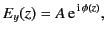(1066)

whereis a constant, and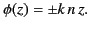(1067)

Note that the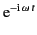time dependence of all wave quantities is taken as read during this investigation. The solution (1068) represents a wave of constant amplitudeand phase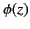. According to Equation (1069), there are, in fact, two independent waves that can propagate through the medium in question. The upper sign corresponds to a wave that propagates vertically upwards, whereas the lower sign corresponds to a wave that propagates vertically downwards. Both waves propagate at the constant phase velocity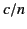.

In general, ifthen the solution to Equation (1067) does not remotely resemble the wave-like solution (1068). However, in the limit that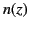is a slowly varying'' function of(exactly how slowly varying is something that will be established later), we expect to recover wave-like solutions. Let us suppose thatis indeed a slowly varying function, and let us try substituting the wave solution (1068) into Equation (1067). We obtain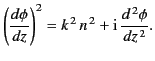(1068)

which is a non-linear differential equation that, in general, is very difficult to solve. However, ifis a constant then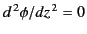. It is, therefore, reasonable to suppose that ifis a slowly varying function then the last term on the right-hand side of the previous equation can be regarded as small. Thus, to a first approximation, Equation (1070) yields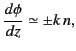(1069)

and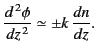(1070)

It is clear from a comparison between Equations (1070) and (1072) thatcan be regarded as a slowly varying function ofas long as its variation length-scale is far longer than the wavelength of the wave. In other words, provided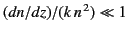.

The second approximation to the solution is obtained by substituting Equation (1072) into the right-hand side of Equation (1070):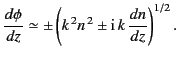(1071)

This gives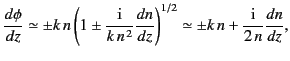(1072)

where use has been made of the binomial expansion. The previous expression can be integrated to give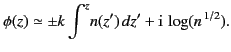(1073)

Substitution of Equation (1075) into Equation (1068) yields the final result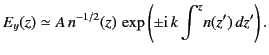(1074)

It follows from Equation (4.183) that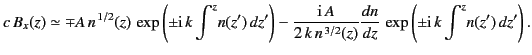(1075)

Note that the second term on the right-hand side of the previous expression is small compared to the first, and can usually be neglected.

Let us test to what extent the expression (1076) is a good solution of Equation (1067) by substituting the former into the left-hand side of the latter. The result is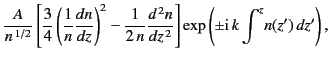(1076)

which must be small compared with either term on the left-hand side of (1067). Hence, the condition for expression (1076) to be a good solution of Equation (1067) becomes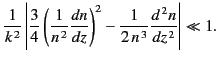(1077)

The solution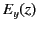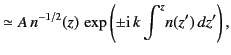(1078)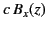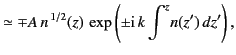(1079)

to the non-uniform wave equations (1063)-(1064) is usually called the WKB solution, in honor of G. Wentzel, H.A. Kramers, and L. Brillouin, who are credited with independently discovering it (in a quantum mechanical context) in 1926. Actually, H. Jeffries wrote a paper on this solution (in a wave propagation context) in 1923. Hence, some people call it the WKBJ solution (or even the JWKB solution). In fact, this solution was first discussed by Liouville and Green in 1837, and again by Rayleigh in 1912. In the following, we refer to (1080)-(1081) as the WKB solution, because this is what it is generally called. However, it should be understand that, in doing so, we are not making any statement as to the credit due to various scientists in discovering this solution.

As is well known, if a propagating electromagnetic wave is normally incident on an interface where the refractive index suddenly changes (for instance, when a light wave propagating in the air is normally incident on a glass slab) then there is generally significant reflection of the wave. However, according to the WKB solution, (1080)-(1081), if a propagating wave is normally incident on a medium in which the refractive index changes slowly along the direction of propagation of the wave then the wave is not reflected at all. This is true even if the refractive index varies very substantially along the path of the wave, as long as it varies slowly. The WKB solution implies that as the wave propagates through the medium its wavelength gradually changes. In fact, the wavelength at positionis approximately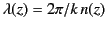. Equations (1080)-(1081) also imply that the amplitude of the wave gradually changes as it propagates. In fact, the amplitude of the electric field component is inversely proportional to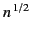, whereas the amplitude of the magnetic field component is directly proportional to. Note, however, that the energy flux in the-direction, which is given by the the Poynting vector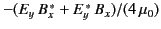, remains constant (assuming thatis predominately real).

Of course, the WKB solution, (1080)-(1081), is only approximate. In reality, a wave propagating into a medium in which the refractive index is a slowly varying function of position is subject to a small amount of reflection. However, it is easily demonstrated that the ratio of the reflected amplitude to the incident amplitude is of order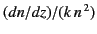. Thus, as long as the refractive index varies on a much longer length-scale than the wavelength of the radiation, the reflected wave is negligibly small. This conclusion remains valid as long as the inequality (1079) is satisfied. There are two main reasons why this inequality might fail to be satisfied. First of all, if there is a localized region in the dielectric medium where the refractive index suddenly changes (i.e., if there is an interface) then (1079) is likely to break down in this region, allowing strong reflection of the incident wave. Secondly, the inequality obviously breaks down in the vicinity of a point where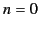. We would, therefore, expect strong reflection of the incident wave from such a point.Next: Reflection Coefficient Up: Wave Propagation in Inhomogeneous Previous: Ionospheric Radio Wave Propagation
Richard Fitzpatrick 2014-06-27